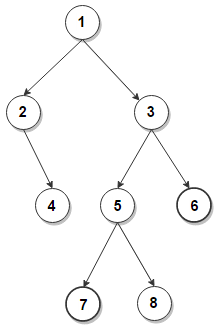# Find the distance between given pairs of nodes in a binary tree

Given a binary tree, determine the distance between given pairs of nodes in it. Distance between two nodes is defined as the number of edges in shortest path from one node from other.

For example, consider below binary tree. The distance between node 7 and node 6 is 3.This problem is a standard application of lowest common ancestor of given nodes. The distance from v to w can be computed as the distance from the root to v, plus the distance from the root to w, minus twice the distance from the root to their lowest common ancestor.

## C++

The time complexity of above solution is O(n) and need O(h) extra space for the call stack where h is the height of the tree.(1 votes, average: 5.00 out of 5)Loading...